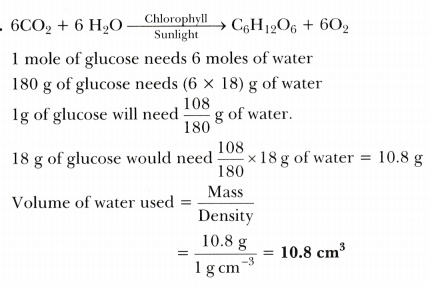# In photosynthesis, 6 molecules of carbon dioxide combine with an equal number of water molecules through a complex series of reactions to give a molecule of glucose having a molecular formula C6H1206

In photosynthesis, 6 molecules of carbon dioxide combine with an equal number of water molecules through a complex series of reactions to give a molecule of glucose having a molecular formula C6H1206. How many grams of water would be required to produce 18 g of glucose? Compute the volume of water so consumed assuming the density of water to be 1 g cm-3.